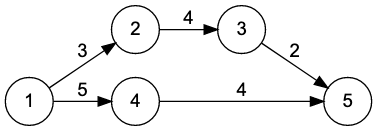시간 제한 메모리 제한 제출 정답 맞은 사람 정답 비율
4 초 2048 MB (추가 메모리 없음) 22 10 10 45.455%

## 문제

After a long time apart, Alice and Bob have reunited. They live in a country with $n$ cities, creatively named city $1$ to city $n$. Bob drove from his home in city $1$ to Alice's home in city $n$.

When Alice asked Bob which path he took, he was stunned to find he didn't remember. Bob is efficient, and drove without stopping, and knows there is no path faster than the one he took. He also has a brand new National Adventuring Company (NAC) TraveLog! Every time Bob drives through a city, the TraveLog records the time between when he left city $1$ and when he arrived in the current city.In the above layout, there are two possible fastest paths Bob can take from city $1$ to city $n$: $1 \to 2 \to 3 \to 5$ or $1 \to 4 \to 5$. Both paths take a total of $9$ units of time. The first path would have a TraveLog of $0, 3, 7, 9$, and the second would have a TraveLog of $0, 5, 9$.

Unfortunately, the memory in Bob's TraveLog is corrupted. Bob thinks that some of the times are gone, and the remaining times are shuffled arbitrarily. Given what remains of the TraveLog, can you reconstruct Bob's path?

## 입력

The first line of input contains three integers $n$ ($2 \le n \le 2 \cdot 10^{5}$), $m$ ($1 \le m \le 3 \cdot 10^{5}$) and \mbox{$d$ ($1 \le d \le n$)}, where $n$ is the number of cities in the country, $m$ is the number of one-way roads between those cities, and $d$ is the number of times remaining in Bob's corrupted TraveLog. The cities are identified by number, $1$ through $n$. Bob lives in city $1$, and Alice lives in city $n$.

Each of the next $m$ lines contains three integers $u$, $v$ ($1 \le u,v \le n, u \ne v$) and $h$ ($1 \le h \le 10^{6}$). Each line describes a one-way road from city $u$ to city $v$ that takes $h$ units of time to traverse. There is guaranteed to be at least one path from city $1$ to city $n$. There may be several roads between any given pair of cities.

Each of the next $d$ lines contains a single integer $t$ ($0 \le t \le 10^{18}$). This is what remains of Bob's TraveLog. Each line is a record of the amount of time Bob took driving from city $1$ to another city on his path. These values are guaranteed to be distinct.

## 출력

The output format depends on the number of paths consistent with Bob's TraveLog.

• If there is no path consistent with Bob's TraveLog, output $0$.
• If multiple paths are consistent with Bob's TraveLog, output $1$.
• Otherwise, on the first line, output the number of cities on Bob's path. On subsequent lines, output the cities Bob visited, one per line, in the order he visited them.

## 예제 입력 1

5 5 2
1 2 3
2 3 4
3 5 2
1 4 5
4 5 4
5
9


## 예제 출력 1

3
1
4
5


## 예제 입력 2

6 8 2
1 2 1
2 3 2
3 6 8
1 4 3
4 5 4
5 6 4
5 2 7
1 6 13
0
3


## 예제 출력 2

1


## 예제 입력 3

2 1 1
1 2 10
5


## 예제 출력 3

0


## 출처• 문제를 만든 사람: Arnav Sastry